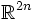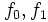Immersions in Euclidean space are isotopic in its square

Suppose$f_0,f_1:M \to \R^n$ are two immersions. Consider$\R^n$ embedded inside$\R^{2n}$. Then, the induced immersions of$f_0, f_1$ in$\R^{2n}$ are isotopic as immersions in$\R^{2n}$.
The key idea of the proof is to swing$f_0$ from the first factor$\R^n$ to the second factor$\R^n$, and then swing back, gradually changing$f_0$ to$f_1$.